# Supervised vs Unsupervised Learning, Explained

The tutorial will start by discussing some foundational concepts and then it will explain supervised and unsupervised learning separately, in more detail.

If you need something specific, just click on the link. The following links will take you to specific sections of the article.

Having said that, if you’re confused about supervised vs unsupervised learning, you’ll probably want to read the whole article from start to finish.

## Supervised vs Unsupervised Learning: A Quick Introduction

If you’re somewhat new to machine learning, you’ve probably heard the terms “supervised” and “unsupervised” learning.

The difference is often confusing to machine learning beginners, and unfortunately, it’s often poorly explained in most textbooks.

Let me quickly explain what these terms mean, and after that, I’ll dive into each topic separately, to go into a little more depth.

### Training Data is Different for Supervised vs Unsupervised Learning

I think that the best way to think about the difference between supervised vs unsupervised learning is to look at the structure of the training data.

In supervised learning, the data has an output variable that we’re trying to predict.

But in a dataset for unsupervised learning, the target variable is absent. There are still “input” variables, but there’s no target.So if we’re doing supervised learning for regression, the training data will have a numeric “target” variable that we’re trying to predict. Or if we’re doing supervised learning for classification, the dataset will have a column that contains the correct label for the row of data.

This, of course, is somewhat simplified. I’m being a little imprecise here for the sake of simplicity.

That being the case, let’s take a look at each of these types of machine learning – supervised learning and unsupervised learning – one at a time.

## Supervised Learning, Explained

As I mentioned above, in Supervised learning, we have a dataset that contains “input” variables and an output variable.

To see this, let’s take a look at a simple example.

Below, we have a dataset.This dataset has a set of input variables. Frequently, in machine learning we talk about these as the “X” variables, and they’re often referred to generally as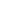,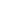,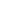. (Keep in mind though, when you work on a specific machine learning problem, these input variables will often have specific names. They are the names of the input variables in your dataset.)

In the machine learning literature, the “input” variables collectively have several different names. Collectively, they are are referred to as features, predictors, or independent variables. Different people use different terminology, but feature, predictor, input variable, and independent variable all essentially mean the same thing.

But when we do supervised learning, the dataset will also have a target variable,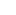.

In supervised learning, this target variable is very important. In fact, in supervised learning, the task for the learning algorithm is to learn how to predicton the basis of the input variables,,, ….

But why do we call it “supervised” learning? The structure of the data in supervised learning actually provides some insight.

### Why We Call It “Supervised” Learning

During the learning process, the target variable,, supervises the learning process.

We feed the training data into the learning algorithm. During the learning process, the algorithm uses the target variableto produce the model.

In supervised learning, the resulting model that can make predictions. For any set of values for the input variables, the model will produce a predicted output that we can call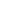.But after the model is built, we can also use the original targetto evaluate the model. To do this, we can compareto.

Ideally, a good machine learning model will make good predictions. If the values inare close to the values in, then it’s probably a good model. But ifis too far away from, then it might not be a good model. Or there might be better model that we could build.

So ultimately, the comparison of the actual valueto the predicted valueprovides feedback which “supervises” the creation of the model. The target variablealso “supervises” the evaluation process in supervised learning.

Now to be clear, there’s a lot of nuance to this once you get into the details. I’m explaining this in a rough, imprecise way to help you understand what’s happening in supervised learning.

But the important thing to understand is that in supervised learning, we have a target variable. And that target variable “supervises” the model building process.

### The Most Popular Supervised Learning Algorithms

Before we move on to unsupervised learning, let’s quickly talk about some common supervised learning algorithms.

In machine learning, many of the most popular and most frequently used techniques are supervised learning algorithms.

For example, all of the following are supervised learning techniques:

• Linear Regression
• Logistic Regression
• Support Vector Machines
• Decision Trees (including Random Forests and Boosted Trees)
• Deep Neural Networks

(Although, there are some versions of deep networks that are unsupervised as well.)

What this means is that as a beginner, you’ll mostly work with supervised learning techniques. In fact, if you’re a beginner, I recommend that you mostly focus on supervised learning first, with 1 or 2 exceptions.

## Un-Supervised Learning, Explained

Now, let’s turn to unsupervised learning.

If you already understand supervised learning, then you should be able to understand what unsupervised learning is by way of comparison.

Let’s start with the data. To me, much like when you’re trying to understand supervised learning, the best place to start when you’re trying to understand unsupervised learning is with the input data.

Similar to supervised learning, in unsupervised learning, our input data has “input” variables,,, ….But in contrast to supervised learning, there’s no supervising output variable in unsupervised learning. The so-called “target” variableis absent from the data. There’s nothing to predict. There isn’t a structured, well-defined output that the learning algorithm can generate.

That being the case, because the target variable is absent, we can’t use supervised learning techniques on such a dataset.

But, we can use unsupervised algorithms on such a dataset.

### Unsupervised learning is often used to find structure in data

So what exactly would we use unsupervised learning for?

Isn’t machine learning about predicting things?

No, not always.

There are some types of problems where prediction is not the goal.

Instead, what’s often the case in unsupervised learning, is that we want to find structure in the data.

To understand this sort of problem, let’s look at a quick example.

### Example of Unsupervised Learning: Clustering

A quintessential example of unsupervised learning is clustering.

There are several types of clustering algorithms, including K-means clustering, hierarchical clustering, and others.

Speaking roughly though, clustering algorithms have the same objective: to identify groups in a dataset.

To understand, let’s look at a visual example.

Let’s say that you have a dataset. And the dataset has only two variables:and. (A “target” variableis completely absent from this dataset.)

Using a scatterplot, you can plot the input variables variables, like this:When you plot these variables as a scatterplot, it should be obvious to you that there’s some structure in this dataset. It’s obvious to a human that there’s some structure here.

But how do we enable a computer to find that structure?

We can use unsupervised learning.

##### Unsupervised Learning Enables a Computer to Find Structure, Automatically

Unsupervised learning provides a set of tools that will enable a computer to identify this structure in a dataset.

So for example, we could use K-means clustering on this data, which is an unsupervised learning technique. By using K-means clustering, a computer could identify a set of “clusters” in this input data. In K-means clustering, “clusters” are groups of observations that are similar to each other.So K-means is a technique that enables a computer to find structure in an input dataset.

Now to be fair, this is really an ultra-simple example. Real world clustering problems typically involve a lot more than two input variables. And the so-called “clusters” are almost never so clearly separated. In real-world clustering problems, you have messy, complex data, as well hard choices about what actually constitutes a “cluster”.

Setting those complexities aside, this should give you a rough idea of what clustering looks like. And this, in turn, should help you understand what unsupervised learning is and why we use it: it’s often used to find structure in data.

#### Note: this is not the same as classification

Now before you get confused, I want to make a point. Clustering like what I showed you above is not the same thing as classification (a type of supervised learning).

In classification, there is a well defined set of possible output classes.

So for example, we might have a dataset where we’re trying to classify rows of data as X’s or O’s. In that case, the possible output classes are well defined: X or O.

Or in a different problem, we might have a dataset where we’re trying to classify dogs and cats. So in that problem, the output classes are well defined: dog or cat.

Classification problems have a well defined set of possible output classes.

However, in clustering, there are not well defined output classes. There’s nothing that we’re trying to predict. There’s just an input dataset, and the clustering algorithm tries to find distinct groups.

### The Most Popular Unsupervised Learning Techniques

Unsupervised learning is somewhat less commonly used, especially by machine learning beginners. Having said that, there are still some important use cases, and a variety of techniques for different tasks.

Broadly, the most common uses for unsupervised learning are:

• dimension reduction
• clustering

For example, Principal Component Analysis (PCA) is an unsupervised learning technique. PCA is one of one of the most important techniques for “dimension reduction” (i.e., reducing the number of dimensions/predictors in a dataset).

And, as mentioned previously, there are several different “clustering” techniques for grouping together rows of data into “clusters”.

That said, a few of the most common unsupervised learning techniques are:

• Principal Component Analysis
• K-Means Clustering
• Hierarchical Clustering

To be clear though, there are also quite a few other unsupervised learning techniques. I’ll leave those for another blog post.

## There Are Also Other Types of Machine Learning

Although supervised learning and unsupervised learning are the two most common categories of machine learning (especially for beginners), there are actually two other machine learning categories worth mentioning: semisupervised learning and reinforcement learning.

### Semisupervised Learning

Semi-supervised learning is somewhat similar to supervised learning.

Remember that in supervised learning, we have a so-called “target” vector,. This contains the output values that we want to predict.

It’s important to remember that in supervised learning learning, the the target variablehas a value for every row.

But in semi-supervised learning, it’s a little different. In semi-supervised learning, there is a target variable. BUT, some of the values are missing.So it’s similar to supervised learning, in the sense that there is a target variable that can supervise the modeling process.

But it’s slightly different in the sense that the target variable has some missing values. This introduces some challenges, and different tools.

### Reinforcement Learning

Reinforcement learning is another category of machine learning techniques.

This is a complicated subject, so I won’t explain this in depth.

But at a high level, here’s what it is:

In reinforcement learning, the learning system can perform certain actions. Favorable actions are rewarded and unfavorable actions are penalized.

Over time, the learning system “learns” the best actions to take by pursuing rewards and avoiding penalties.

Although this is a very complicated subject that’s beyond the scope of this blog post, you should note that reinforcement learning has some interesting applications. For example, machine learning systems that learn to play video games are frequently built using reinforcement learning. The AlphaGo system (built by DeepMind) also incorporated reinforcement learning.

Again though reinforcement learning is a more advanced topic. So if you’re a relative beginner in machine learning, you should stick to supervised and unsupervised learning.

Do you still have questions about supervised vs unsupervised learning?

If you have specific questions or you think that there’s something I haven’t explained sufficiently, please leave your question in the comments section at the bottom of the page.

## There’s a lot more to learn

This article should have given you a good overview of supervised vs unsupervised learning.

But if you really want to master machine learning there is a lot more to learn. You’ll need to understand:

• regression vs classification
• model building
• model evaluation
• deployment

…. as well as a variety of specific supervised and unsupervised learning techniques.

If you’re interested in learning more about machine learning, then sign up for our email list. Through this year and into the foreseeable future, we’ll be posting detailed tutorials about different parts of the machine learning workflow.

We plan to publish detailed tutorials about the different machine learning techniques, like linear regression, logistic regression, decision trees, neural networks, and more.

So if you want to master machine learning, then sign up for our email list. When you sign up, we’ll send our new tutorials directly to your inbox as soon as they’re published.

Joshua Ebner

Joshua Ebner is the founder, CEO, and Chief Data Scientist of Sharp Sight. Prior to founding the company, Josh worked as a Data Scientist at Apple. He has a degree in Physics from Cornell University.

### 7 thoughts on “Supervised vs Unsupervised Learning, Explained”

1. Very good explanation! Thanks the author, you clearly defined the difference between the supervised and unsupervised ML which help to understand why my dataset which has some logic structure inside won’t produce good prediction.

• Great to hear that you liked the explanation and found it useful.

2. Great introduction to the topic! I am a total beginner so I’m wondering how does deep learning fit into all these? Is deep learning a subset of unsupervised learning?

As AlphaGo was created by DeepMind, using reinforcement learning in the process, is RL then also considered a form of deep learning?

• Deep learning is a bit of a special beast. It’s more like a toolkit for doing machine learning … a toolkit that involves “deep” networks of artificial neurons.

So there are deep learning techniques that qualify for all of the different categories … there are deep learning techniques for supervised learning, unsupervised learning, and reinforcement learning.

Again, deep learning is a bit of a special topic, and a more advanced topic. I’ll definitely be posting tutorials about deep learning at some point (probably later this year and next year). In the meantime, try to focus on the fundamentals.

3. As usual, the clarity and fluidity of your explanations are a breath of fresh air and so bludy helpful!
— non-technical, non-STEM fan of your blog